•5星
1KB weixin_42696333 2021-09-10 17:11:48
•5星
8.51MB GZXGYZ 2021-08-16 10:12:36
•5星
162KB ailemony 2020-12-08 22:44:13
•5星
387KB weixin_44510615 2021-06-23 18:04:15
•5星
1.82MB xiaolong1126626497 2021-05-26 21:00:57
•5星
570.7MB ECHOSON 2021-05-24 10:21:12
•5星
163.17MB weixin_40632177 2020-12-14 21:24:12
•5星
401KB m0_52957036 2020-12-16 06:20:16
•5星
1.77MB m0_52957036 2020-04-19 17:01:47
• 582KB wujiang0156 2020-05-21 13:16:19
• 树的双亲表示法，孩子表示法以及孩子兄弟表示法 数据结构与算法分析 二叉树 双亲表示法 C语言

文章目录树的双亲表示法树的孩子表示法树的孩子兄弟表示法 顺序存储和链式存储，本节来学习如何存储具有普通树结构的数据。 树的双亲表示法 双亲表示法采用顺序表（也就是数组）存储普通树，其实现的核心思想是：...

文章目录

如下图所示，这是一棵普通的树，该如何存储呢？通常，存储具有普通树结构数据的方法有 3 种：
双亲表示法；
孩子表示法；
孩子兄弟表示法；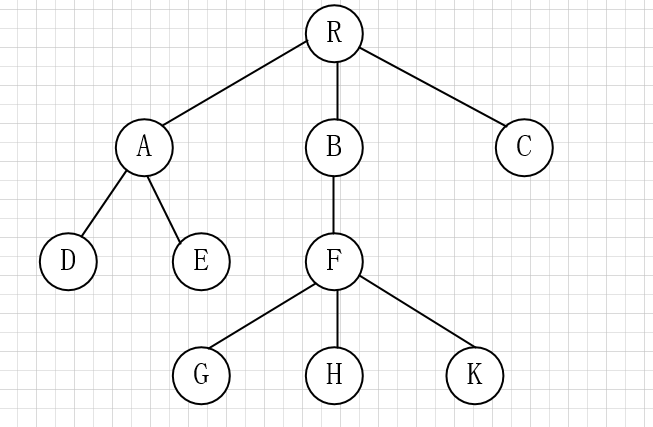图1

树的双亲表示法

双亲表示法采用顺序表（也就是数组）存储普通树，其实现的核心思想是：顺序存储各个节点的同时，给各节点附加一个记录其父节点位置的变量
注意，根节点没有父节点（父节点又称为双亲节点），因此根节点记录父节点位置的变量通常置为 -1。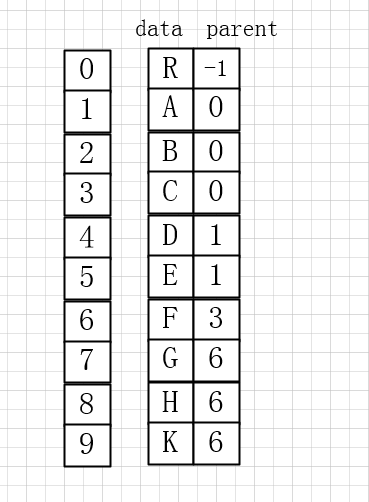图2
双亲表示法存储普通树代码

/*
* @Description: 树的双亲表示法
* @Version: V1.0
* @Autor: Carlos
* @Date: 2020-05-21 14:41:32
* @LastEditors: Carlos
* @LastEditTime: 2020-06-01 22:12:34
*/
#include<stdio.h>
#include<stdlib.h>
//宏定义树中结点的最大数量
#define MAX_SIZE 20
//宏定义树结构中数据类型
typedef char ElemType;
//结点结构
typedef struct Snode
{
//树中结点的数据类型
ElemType data;
//结点的父结点在数组中的位置下标
int parent;
}PNode;
//树结构
typedef struct
{
//存放树中所有结点
PNode tnode[MAX_SIZE];
//结点个数
int n;
}PTree;
/**
* @Description: 节点初始化
* @Param: PTree tree 结构体变量
* @Return: PTree 结构体地址
* @Author: Carlos
*/
PTree InitPNode(PTree tree)
{
int i,j;
char ch;
printf("请输入节点个数:\n");
scanf("%d",&(tree.n));

printf("请输入结点的值其双亲位于数组中的位置下标:\n");
for(i=0; i<tree.n; i++)
{
fflush(stdin);
scanf("%c %d",&ch,&j);
tree.tnode[i].data = ch;
tree.tnode[i].parent = j;
}
return tree;
}
/**
* @Description: 查找树中指定节点
* @Param: PTree tree 结构体变量
* @Return: 无
* @Author: Carlos
*/
void FindParent(PTree tree)
{
char a;
int isfind = 0;
printf("请输入要查询的结点值:\n");
fflush(stdin);
scanf("%c",&a);
for(int i =0;i<tree.n;i++){
if(tree.tnode[i].data == a){
isfind=1;
//找到父节点的下标数值
break;
}
}
if(isfind == 0){
printf("树中无此节点");
}
}

int main()
{
PTree tree;
tree = InitPNode(tree);
FindParent(tree);
return 0;
}

树的孩子表示法

孩子表示法存储普通树采用的是 “顺序表+链表” 的组合结构，其存储过程是：从树的根节点开始，使用顺序表依次存储树中各个节点，需要注意的是，与双亲表示法不同，孩子表示法会给各个节点配备一个链表，用于存储各节点的孩子节点位于顺序表中的位置。
如果节点没有孩子节点（叶子节点），则该节点的链表为空链表。
例如，使用孩子表示法存储左图中的普通树，则最终存储状态如右图所示：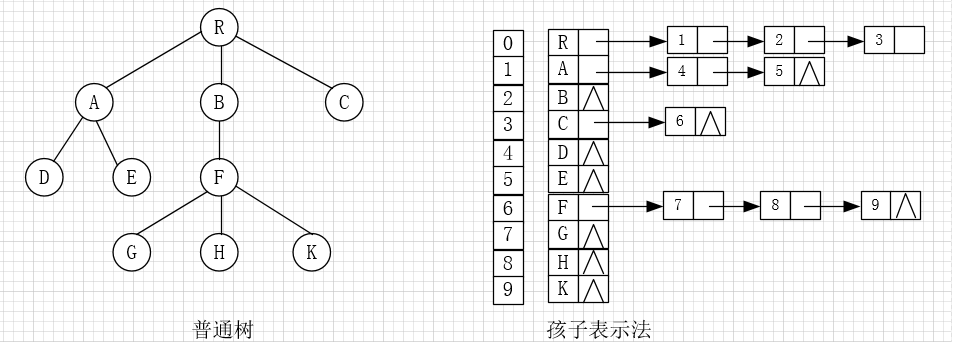图3

/*
* @Description: 树的孩子表示法。三部分构成，链表，节点，树
* @Version:
* @Autor: Carlos
* @Date: 2020-05-21 14:59:47
* @LastEditors: Carlos
* @LastEditTime: 2020-06-01 22:47:38
*/

#include<stdio.h>
#include<stdlib.h>
#define MAX_SIZE 20
#define TElemType char
typedef struct CTNode{
//链表中每个结点存储的不是数据本身，而是数据在数组中存储的位置下标！！
int child;
struct CTNode * next;
}ChildPtr;
typedef struct {
//结点的数据类型
TElemType data;
//孩子链表的头指针
ChildPtr* firstchild;
}CTBox;
typedef struct{
//存储结点的数组
CTBox nodes[MAX_SIZE];
//结点数量和树根的位置
int n,r;
}CTree;
/**
* @Description: 孩子表示法存储普通树
* @Param: CTree tree 树的结构体变量
* @Return: CTree tree 结构体变量
* @Author: Carlos
*/
CTree InitTree(CTree tree){
printf("输入节点数量：\n");
scanf("%d",&(tree.n));
for(int i=0;i<tree.n;i++){
printf("输入第 %d 个节点的值：\n",i+1);
fflush(stdin);
scanf("%c",&(tree.nodes[i].data));
tree.nodes[i].firstchild=(ChildPtr*)malloc(sizeof(ChildPtr));
tree.nodes[i].firstchild->next=NULL;

printf("输入节点 %c 的孩子节点数量：\n",tree.nodes[i].data);
int Num;
scanf("%d",&Num);
if(Num!=0){
//带头结点的链表
ChildPtr * p = tree.nodes[i].firstchild;
for(int j = 0 ;j<Num;j++){
ChildPtr * newEle=(ChildPtr*)malloc(sizeof(ChildPtr));
newEle->next=NULL;
printf("输入第 %d 个孩子节点在顺序表中的位置",j+1);
scanf("%d",&(newEle->child));
p->next= newEle;
p=p->next;
}
}
}
return tree;
}
/**
* @Description:查找节点
* @Param: CTree tree 树的结构体，char a 要查找的节点
* @Return: 无
* @Author: Carlos
*/
void FindKids(CTree tree,char a){
for(int i=0;i<tree.n;i++){
if(tree.nodes[i].data==a){
ChildPtr * p=tree.nodes[i].firstchild->next;
while(p){
//打印所有孩子节点 p->child 孩子节点在数组中的位置
printf("%c ",tree.nodes[p->child].data);
p=p->next;
}
break;
}
}
printf("此节点为叶子节点");
}
}

int main()
{
CTree tree;
tree = InitTree(tree);
//默认数根节点位于数组notes处
tree.r=0;
printf("找出节点 F 的所有孩子节点：");
FindKids(tree,'F');
return 0;
}

树的孩子兄弟表示法

树结构中，位于同一层的节点之间互为兄弟节点。例如，图1中的普通树中，节点 A、B 和 C 互为兄弟节点，而节点 D、E 和 F 也互为兄弟节点。
孩子兄弟表示法，采用的是链式存储结构，其存储树的实现思想是：从树的根节点开始，依次用链表存储各个节点的孩子节点和兄弟节点
因此，该链表中的节点应包含以下 3 部分内容：
节点的值；
指向孩子节点的指针；
指向兄弟节点的指针；

版权声明：本文为博主原创文章，遵循 CC 4.0 BY-SA 版权协议，转载请附上原文出处链接和本声明。
本文链接：https://blog.csdn.net/qq_16933601/article/details/106483088图4
用 C 语言代码表示节点结构为：

#define ElemType char
typedef struct CSNode{
ElemType data;
struct CSNode * firstchild,*nextsibling;
}CSNode,*CSTree;

以图1为例，使用孩子兄弟表示法进行存储的结果如下图所示: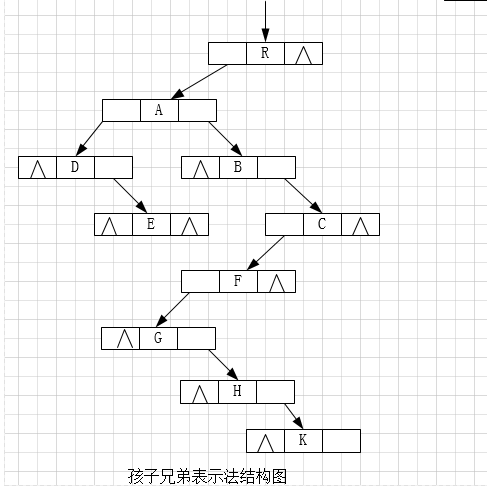图5
由图5可以看到，节点 R 无兄弟节点，其孩子节点是 A；节点 A 的兄弟节点分别是 B 和 C，其孩子节点为 D，依次类推。
实现上图中的 C 语言实现代码也很简单，根据图中链表的结构即可轻松完成链表的创建和使用，因此不再给出具体代码。
接下来观察图 1 和图 5。图 1 为原普通树，图5 是由图 1 经过孩子兄弟表示法转化而来的一棵树，确切地说，图5是一棵二叉树。因此可以得出这样一个结论，即通过孩子兄弟表示法，任意一棵普通树都可以相应转化为一棵二叉树，换句话说，任意一棵普通树都有唯一的一棵二叉树于其对应。
因此，孩子兄弟表示法可以作为将普通树转化为二叉树的最有效方法，通常又被称为"二叉树表示法"或"二叉链表表示法"。

展开全文qq_16933601 2020-06-12 14:43:11
• 树的孩子兄弟表示法完整代码实现 数据结构 c语言 孩子兄弟表示法 树的存储结构

孩子兄弟表示法 孩子兄弟链存储结构的类型声明 typedef struct CSNode { ElemType data; //数据域 struct CSNode *firstchild,*nextsibling; //第一个孩子和右兄弟指针 }CSNode,*CSTree; 孩子兄弟链存储结构是为每...

孩子兄弟表示法

孩子兄弟链存储结构的类型声明

typedef struct CSNode
{
ElemType data; //数据域
struct CSNode *firstchild,*nextsibling; //第一个孩子和右兄弟指针
}CSNode,*CSTree;

• 孩子兄弟链存储结构是为每个结点设计3个域。即一个数据元素域、一个指向该结点的左边第一个孩子结点(长子)的指针域、一个指向该结点的下一个兄弟结点的指针域。由于树的孩子兄弟链存储结构固定有两个指针域，并且这两个指针是有序的(即兄弟域和孩子域不能混淆)，所以孩子兄弟链存储结构实际上是把该树转换为二又树的存储结构。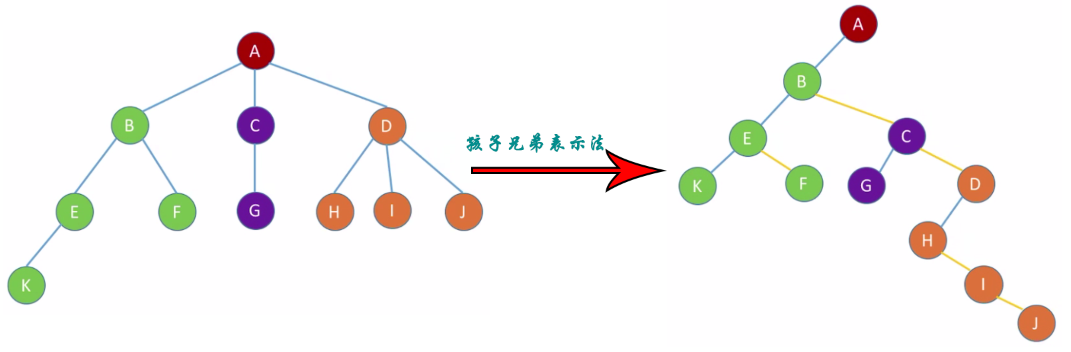• 优点：可方便地实现树和二又树的相互转换。
• 缺点：从当前结点查找双亲结点比较麻烦，需要从树的根结点开始逐个结点比较查找。

代码实现

#include<stdio.h>
#include<malloc.h>
typedef char ElemType;
#define MaxSize 15
typedef struct CSNode
{
ElemType data;
struct CSNode* firstchild, * nextsibling;
}CSNode, * CSTree;
typedef struct
{
CSNode* data[MaxSize]; //存放栈中的数据元素
int top; //存放栈顶指针
}SqStack; //顺序栈类型

void InitStack(SqStack*& s) //初始化栈
{
s = (SqStack*)malloc(sizeof(SqStack)); //分配一个顺序栈空间，首地址存放在s中
if (s == NULL) printf("内存分配不成功！\n");
s->top = -1; //栈顶指针置为-1
}

bool EmptyStack(SqStack* s) //判断栈是否为空
{
return s->top == -1;
}

bool Push(SqStack*& st, CSNode*b) //进栈
{
if (st->top == MaxSize - 1) return false; //栈满的情况，即栈上溢出
st->data[++st->top] = b; //栈顶指针先增1，再将元素放在栈顶指针处
return true;
}

bool Pop(SqStack*& st, CSNode*&b) //出栈
{
if (st->top == -1) return false; //栈空的情况，即栈下溢出
b = st->data[st->top--]; //先取栈顶元素，再使栈顶指针减1
return true;
}

bool GetTop(SqStack* st, CSNode*&b) //取栈顶元素
{
if (st->top == -1) return false; //栈空的情况，即栈下溢出
b = st->data[st->top]; //取栈顶元素
return true;
}

void DestroyStack(SqStack*& s) //销毁栈
{
free(s);
}

void InitCSTree(CSTree t) //构造空树(初始化树)
{
t = NULL;
}

void ClearCSTree(CSTree t) //清空树
{
if (t)
{
if (t->firstchild) ClearCSTree(t->firstchild);
if (t->nextsibling) ClearCSTree(t->nextsibling);
free(t); //释放根结点
t = NULL;
}
}

void DestoryCSTree(CSTree t) //销毁树
{
//此存储结构二叉树无法销毁
}

bool EmptyCSTree(CSTree t) //判断树是否为空
{
return t == NULL;
}

bool CreateCSTree(CSTree &t) //按先序序列构造树
{
char ch;
scanf("%c", &ch);
if (ch == '#') t = NULL;
else
{
t = (CSNode*)malloc(sizeof(CSNode));
if (t == NULL) return false; //分配失败
t->data = ch;
CreateCSTree(t->firstchild);
CreateCSTree(t->nextsibling);
}
return true;
}

int CSTreeDegree(CSTree t)
{
int i = 0, j = 0, max = -1, temp;
CSTree Q; //临时存放各结点
if (t != NULL)
{
max = 0;
Q[j++] = t->firstchild;
while (i < j) //按层序遍历
{
temp = 0;
while (Q[i])
{
temp++;
if (Q[i]->firstchild) Q[j++] = Q[i]->firstchild; //存储有孩子的结点
Q[i] = Q[i]->nextsibling; //统计本层结点个数
}
if (temp > max) max = temp;
i++;
}
}
return max;
}

int CSTreeDepth(CSTree t) //返回树的深度
{
int row = 0, max;
SqStack *st;
CSTree temp;
if (t != NULL)
{
InitStack(st);
Push(st, t);
row = max = 1;
while (!EmptyStack(st))
{
GetTop(st, temp);
while (temp->firstchild)
{
Push(st, temp->firstchild);
max++;
if (row < max) row = max;
GetTop(st, temp);
}
Pop(st, temp);
if (temp->nextsibling) Push(st, temp->nextsibling);
else
{
while (!EmptyStack(st))
{
Pop(st, temp);
max--;
if (temp->nextsibling)
{
Push(st, temp->nextsibling);
break;
}
}
}
}
}
return row;
}

ElemType CSRoot(CSTree t) //返回树的根结点值
{
if (t != NULL) return t->data;
else return '\0';
}

ElemType CSTreeValue(CSTree t, int i) //返回树中第i个结点值(按层序计数)
{
int j = 0, k= 0, count = 0;
CSTree Q;
if (t != NULL && i > 0)
{
Q[k++] = t;
while (j < k)
{
while (Q[j])
{
count++;
if (count == i) return Q[j]->data;
if (Q[j]->firstchild) Q[k++] = Q[j]->firstchild;
Q[j] = Q[j]->nextsibling;
}
}
j++;
}
return '\0';
}

CSTree OrderCS(CSTree t, ElemType e) //返回指向结点e的指针，NULL代表无此结点
{
int i = 0, j = 0, count = 0;
CSTree Q;
if (t != NULL)
{
Q[j++] = t;
while (i < j)
{
while (Q[i] && Q[i]->data != e)
{
if (Q[i]->firstchild) Q[j++] = Q[i]->firstchild;
Q[i] = Q[i]->nextsibling;
}
if (Q[i] && Q[i]->data == e) return Q[i];
i++;
}
}
return NULL;
}

bool CSAssign(CSTree t, ElemType e, ElemType value) //替换结点e的值为value
{
CSNode* p = OrderCS(t, e);
if (p != NULL) //找到了e
{
p->data = value;
return true;
}
return false;
}

ElemType CSChildValue(CSTree t, ElemType e, int order) //返回结点e的第order个孩子的值(从左至右计数)
{
int i = 0, j = 0, count = -1;
CSTree Q;
if (t != NULL)
{
Q[j++] = t;
while (i < j)
{
while (Q[i] && Q[i]->data != e)
{
if (Q[i]->firstchild) Q[j++] = Q[i]->firstchild;
Q[i] = Q[i]->nextsibling;
}
if (Q[i] && Q[i]->data == e) break;
i++;
}
if (i < j)
{
count = 0;
if (Q[i]->firstchild)
{
Q[i] = Q[i]->firstchild;
while (Q[i])
{
count++;
if (count == order) return Q[i]->data;
Q[i] = Q[i]->nextsibling;
}
}
}
}
return '\0';
}

ElemType SiblingCS(CSTree t, ElemType e, int mark) //返回元素e的左(右)兄弟，mark标记左右
{
int i = 0, j = 0, m = 0, n = 0;
CSTree Q;
ElemType key = {};
if (t != NULL && t->data != e)
{
Q[j++] = t;
key[n++] = t->data;
while (i < j)
{
while (Q[i])
{
if (Q[i]->firstchild)
{
Q[j++] = Q[i]->firstchild;
key[n++] = Q[i]->firstchild->data;
}
if (mark == 0)
{
if (Q[i]->data == e && Q[i]->data == key[m]) return '\0';
if (Q[i]->nextsibling && Q[i]->nextsibling->data == e) return Q[i]->data;
}
else
{
if (Q[i]->data == e && Q[i]->nextsibling) return Q[i]->nextsibling->data;
}
Q[i] = Q[i]->nextsibling;
}
i++;
m++;
}
}
return '\0';
}

CSNode* CSChildSeat(CSTree t, ElemType e, int i) //返回树中结点e的第i个孩子(层序计数)的指针
{
ElemType temp;
CSNode* p = NULL;
temp = CSChildValue(t, e, i);
if (temp) p = OrderCS(t, temp);
return p;
}

int ChildCount(CSTree t, ElemType p) //返回结点p的孩子结点(子树)个数，返回负数代表结点p不存在
{
int i = 0, j = 0, count = -1;
CSTree Q;
if (t)
{
Q[j++] = t;
while (i < j)
{
while (Q[i] && Q[i]->data != p)
{
if (Q[i]->firstchild) Q[j++] = Q[i]->firstchild;
Q[i] = Q[i]->nextsibling;
}
if (Q[i] && Q[i]->data == p) break;
i++;
}
if (i < j) //找到了p
{
count = 0;
if (Q[i]->firstchild)
{
Q[i] = Q[i]->firstchild;
while (Q[i])
{
count++;
Q[i] = Q[i]->nextsibling;
}
}
}
}
return count;
}

bool InsertCSTree(CSTree T, ElemType e, int i, CSTree t) //将树t插入为树t中e结点的第i棵子树(层序计数)，i=0定义为最后一棵子树
{
int j, k0;
CSNode* p, * q;
k0 = ChildCount(t, e);
if (k0 < 0 || i<0 || i>k0 + 1) return false;
if (i == 0) j = k0 + 1;
else j = i;
if (j == 1)
{
p = OrderCS(t, e);
t->nextsibling = p->firstchild;
p->firstchild = t;
}
else {
q = CSChildSeat(t, e, j - 1);
t->nextsibling = q->nextsibling;
q->nextsibling = t;
}
return true;
}

bool DeleteCSTree(CSTree t, ElemType e, int i) //删除树中e结点的第i棵子树
{
ElemType tmp;
CSNode *p, *q;
if (i == 1)
{
p = OrderCS(t, e);
if (!p) return false;
q = p->firstchild->nextsibling;
p->firstchild->nextsibling = NULL;
ClearCSTree(p->firstchild);
p->firstchild = q;
}
else
{
p = CSChildSeat(t, e, i - 1);
if (!p) return false;
q = p->nextsibling->nextsibling;
p->nextsibling->nextsibling = NULL;
ClearCSTree(p->nextsibling);
p->nextsibling = q;
}
return true;
}

void LevelOrder(CSTree t) //层序遍历树
{
int i = 0, j = 0;
CSTree Q;
if (t) Q[j++] = t;
while (i < j)
{
while (Q[i])
{
printf("%c ", Q[i]->data);
if (Q[i]->firstchild) Q[j++] = Q[i]->firstchild;
Q[i] = Q[i]->nextsibling;
}
i++;
}
}

void PreOrder(CSTree t) //前序遍历树
{
if (t)
{
printf("%c ", t->data);
PreOrder(t->firstchild);
PreOrder(t->nextsibling);
}
}

void InOrder(CSTree t) //中序遍历树
{
if (t)
{
InOrder(t->firstchild);
printf("%c ", t->data);
InOrder(t->nextsibling);
}
}

int main()
{
CSNode* t = NULL;
InitCSTree(t);
printf("输入：");
CreateCSTree(t);
printf("\n前序遍历树：");
PreOrder(t);
printf("\n中序遍历树：");
InOrder(t);
printf("\n层次遍历树：");
LevelOrder(t);
printf("\n树的根结点为：%c", CSRoot(t));
printf("\n树的度为：%d", CSTreeDegree(t));
printf("\n树共有：%d层", CSTreeDepth(t));
return 0;
}

运行结果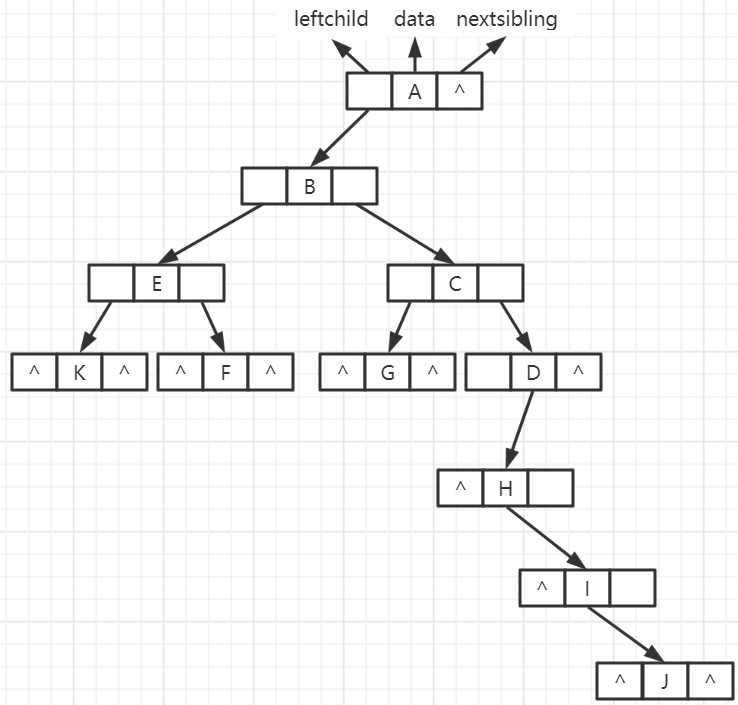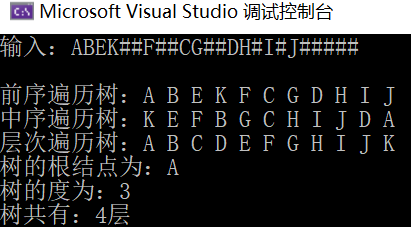展开全文weixin_42071236 2021-08-31 17:13:28
• 前面讲解了存储普通树的双亲表示法和孩子表示法，本节来讲解最后一种常用方法——孩子兄弟表示法。图 1 普通树示意图树结构中，位于同一层的节点之间互为兄弟节点。例如，图 1 的普通树中，节点 A、B 和 C 互为兄弟...

前面讲解了存储普通树的双亲表示法和孩子表示法，本节来讲解最后一种常用方法——孩子兄弟表示法。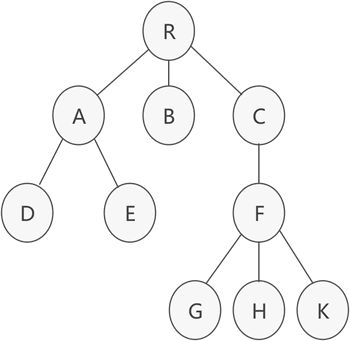图 1 普通树示意图

树结构中，位于同一层的节点之间互为兄弟节点。例如，图 1 的普通树中，节点 A、B 和 C 互为兄弟节点，而节点  D、E 和 F 也互为兄弟节点。

孩子兄弟表示法，采用的是链式存储结构，其存储树的实现思想是：从树的根节点开始，依次用链表存储各个节点的孩子节点和兄弟节点。

因此，该链表中的节点应包含以下 3 部分内容(如图 2 所示)：

节点的值；

指向孩子节点的指针；

指向兄弟节点的指针；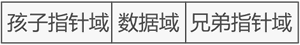图 2 节点结构示意图

用 C 语言代码表示节点结构为：

#define ElemType char typedef struct CSNode{ ElemType data; struct CSNode * firstchild,*nextsibling; }CSNode,*CSTree;

以图 1 为例，使用孩子兄弟表示法进行存储的结果如图 3 所示: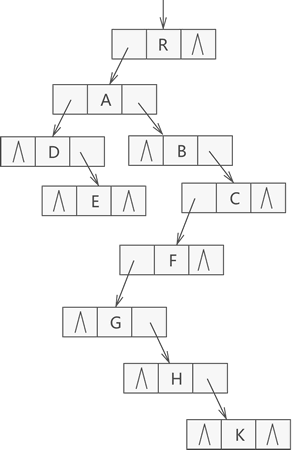图 3 孩子兄弟表示法示意图

由图 3 可以看到，节点 R 无兄弟节点，其孩子节点是 A；节点 A 的兄弟节点分别是 B 和 C，其孩子节点为 D，依次类推。

实现图 3 中的 C 语言实现代码也很简单，根据图中链表的结构即可轻松完成链表的创建和使用，因此不再给出具体代码。

接下来观察图 1 和图 3。图 1 为原普通树，图 3 是由图 1 经过孩子兄弟表示法转化而来的一棵树，确切地说，图 3 是一棵二叉树。因此可以得出这样一个结论，即通过孩子兄弟表示法，任意一棵普通树都可以相应转化为一棵二叉树，换句话说，任意一棵普通树都有唯一的一棵二叉树于其对应。

因此，孩子兄弟表示法可以作为将普通树转化为二叉树的最有效方法，通常又被称为"二叉树表示法"或"二叉链表表示法"。

展开全文weixin_31191387 2021-05-21 02:04:29
• 分别是 双亲表示法 孩子表示法 孩子兄弟表示法 如果现在是这种数据关系; 双亲表示法: 孩子表示法： 孩子兄弟表示法： 感觉最重要的逻辑关系一看图就知道了，关键在于怎么定义其中的数据： 双亲表示法...

今天复习书的存储结构中的表示法

分别是   双亲表示法  孩子表示法 孩子兄弟表示法

如果现在是这种数据关系;双亲表示法: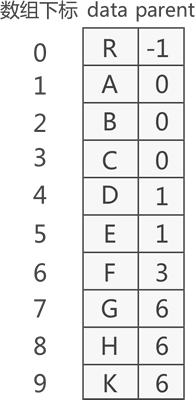孩子表示法：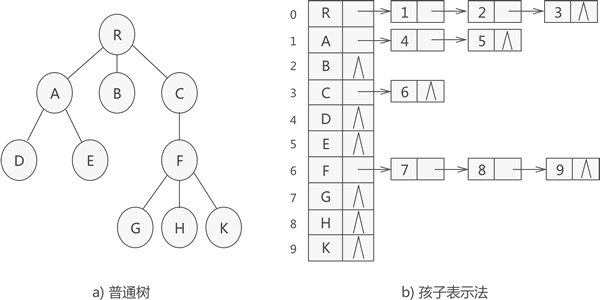孩子兄弟表示法：感觉最重要的逻辑关系一看图就知道了，关键在于怎么定义其中的数据：

双亲表示法定义：

#define MAX_SIZE 100//宏定义树中结点的最大数量
typedef char ElemType;//宏定义树结构中数据类型
typedef struct Snode{
TElemType data;//树中结点的数据类型
int parent;//结点的父结点在数组中的位置下标
}PTNode;
typedef struct {
PTNode tnode[MAX_SIZE];//存放树中所有结点
int n;//根的位置下标和结点数
}PTree;

孩子表示法：

//孩子表示法
typedef struct CTNode{
int child;//链表中每个结点存储的不是数据本身，而是数据在数组中存储的位置下标
struct CTNode * next;
}ChildPtr;
typedef struct {
TElemType data;//结点的数据类型
ChildPtr* firstchild;//孩子链表的头指针
}CTBox;
typedef struct{
CTBox nodes[MAX_SIZE];//存储结点的数组
int n,r;//结点数量和树根的位置
}CTree;

孩子兄弟表示法：

#define ElemType char
typedef struct CSNode{
ElemType data;
struct CSNode * firstchild,*nextsibling;
}CSNode,*CSTree;

展开全文zhuzhenchen 2020-07-10 20:17:35
• vener_ 2020-09-30 16:44:01
• 《数据结构》-树(孩子兄弟表示法) 孩子兄弟表示法 二叉树

tyustli 2021-07-25 21:44:18
• weixin_43960370 2020-05-16 17:26:40
• 树的孩子兄弟表示法C++代码实现 c++ 链表 数据结构

BarbequeBBQ 2021-10-08 20:53:03
• weixin_45895026 2020-01-22 12:23:20
• 编程求以孩子兄弟表示法存储的森林的叶子结点数☆ 二叉树 数据结构 孩子兄弟表示法

qq_36790381 2021-04-01 13:54:52
• 数据结构——N叉树-孩子兄弟表示法|理解and实现N叉树的孩子兄弟表示法|树转化为二叉树 数据结构

weixin_46096297 2021-01-12 20:05:15
• 森林的孩子兄弟表示法 森林的孩子兄弟表示法

qq_42717239 2019-05-06 13:58:35
• 【数据结构·考研】树的孩子兄弟表示法 二叉树 数据结构 c++ dfs bfs

cjw838982809 2020-09-03 23:53:35
• So_cute_SJM 2020-02-02 19:40:01
• 2KB q123456789098 2012-06-17 06:47:01
• 采用孩子兄弟表示法建立一棵树 c语言 数据结构

tygmc 2020-05-24 11:22:10
• 树的孩子兄弟表示法（C/C++） 树结构 数据结构 c++ c语言

Ivan_47 2020-12-17 22:48:25
• qq_25775935 2020-04-15 12:39:13
• zhang911007xing 2017-06-06 03:43:32
• chengou5332 2019-08-10 21:22:00
• lfb637 2017-11-11 17:12:37
• 树的双亲表示法 孩子表示法 孩子兄弟表示法 数据结构与算法

qq_43735092 2019-08-12 10:01:29
• 数据结构——树——孩子兄弟表示法 数据结构

weixin_43945471 2021-01-20 10:38:18
• qq_41017546 2020-03-04 15:43:44
• 常用数据结构之树的双亲_孩子_孩子兄弟表示法 数据结构

gaobing1993 2020-10-03 17:35:11
• vener_ 2020-10-21 16:12:34...